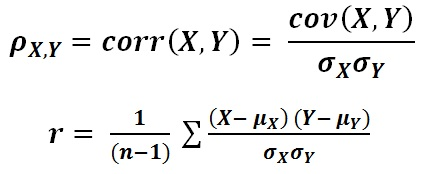## Correlation Coefficient Calculator

 Enter Value of X= 1,2,4,5,8Enter the Numbers with Comma separated(,) Enter Value of Y= 5,20,40,80,100 Enter the Numbers with Comma separated(,)
 Result: No.of Inputs Correlation Coefficient XMean(µx) YMean(µy) σx σy

The Correlation Coefficient Calculator to find the statistical relationship of two sets of date, it is for data analysis how strong the correlation of two quantities is.

The famous measure of dependence is Pearson's correlation, Give two data sets μx and μy, then σx and σy represents the standard deviation of the data set X and Y respectively

### Correlation Coefficient formulaForm the formula, we know if the correlation is high, r lies between 0.9 and 1.0

For example, if μx = (1,2,4,5,8), μy = (5,20,40,80,100), Then Correlation Coefficient r = 0.9684, = 4, = 49, =2.7386, = 40.0625.

Thinkcalculator.com provides you helpful and handy calculator resources.